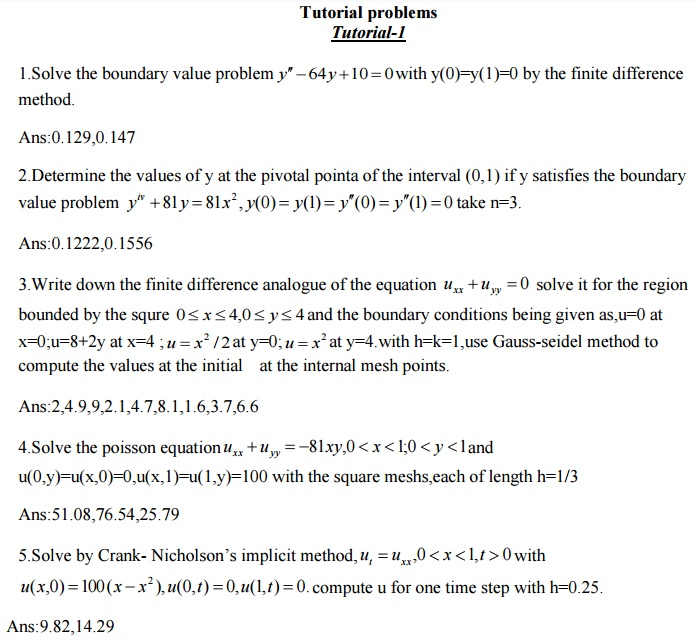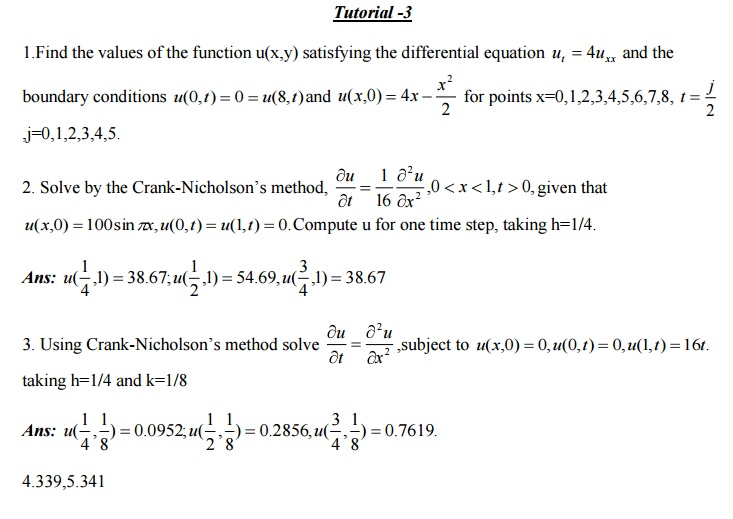Home | | Statistics and Numerical Methods | Important Short Objective Question and Answers: Mathematics (maths) - Boundary Value Problems In Ordinary And Partial Differential Equations

# Important Short Objective Question and Answers: Mathematics (maths) - Boundary Value Problems In Ordinary And Partial Differential Equations

Mathematics (maths) - Boundary Value Problems In Ordinary And Partial Differential Equations - Important Short Objective Question and Answers: Mathematics (maths) - Boundary Value Problems In Ordinary And Partial Differential Equations

1.What is the error for solving Laplace and Poisson’s equations by finite difference method?

Sol:

The error in replacing by the difference expression is of the order . Since h=k, the error in replaing by the difference expression is of the order .2. Define a difference quotient.

Sol:

A difference quotient is the quotient obtained by dividing the difference between two values of a function by the difference between two corresponding values of the independent variable.

3. Why is Crank  Nicholson’s scheme called an implicit scheme?

Sol:

The Schematic representation of crank Nicholson method is shown below.

The solution value at any point (i,j+1) on the (j +1)th level is dependent on the solution values at the neighboring points on the same level and on three values on the j th level. Hence it is an implicit method.

4. What are the methods to solve second order boundary-value problems?

Sol:

(i)Finite difference method (ii)Shooting method.

5. What is the classification of one dimensional heat flow equation.

Sol:

One dimensional heat flow equation isHere A=1,B=0,C=0

B2 −4AC = 0

Hence the one dimensional heat flow equation is parabolic.

6. 6. State Schmidt’s explicit formula for solving heat flow equation

Sol:  ---- ----

7.  Write an explicit formula to solve numerically the heat equation (parabolic equation)

Sol:

--------- ----------

x and k is the space in the time direction).

The above formula is a relation between the function values at the two levels j+1 and j and is called a two level formula. The solution value at any point (i,j+1) on the (j+1)th level is expressed in terms of the solution values at the points (i-1,j),(i,j) and (i+1,j) on the j th level.Such a method is called explicit formula. the formula is geometrically represented below.

8. State the condition for the equation to be

(i) elliptic,(ii)parabolic(iii)hyperbolic when A,B,C are functions of x and y

Sol:

The equation is elliptic if (2B2 ) −4AC < 0

(i.e) B2 −AC < 0. It is parabolic if B2 −AC = 0 and hyperbolic if B2−4AC > 0

9. Write a note on the stability and convergence of the solution of the difference

equation corresponding to the hyperbolic equation    .

Sol:

For ,λ= the solution of the difference equation is stable and coincides with the solution of the differential equation. For λ> ,the solution is unstable.For λ<  ,the solution is stable but not convergent.10.  State the explicit scheme formula for the solution of the wave equation.

Sol:

The formula to solve numerically the wave equation =0 is

The schematic representation is shown below.

The solution value at any point (i,j+1) on the ( j +1)th level is expressed in terms of solution values on the previous j and (j-1) levels (and not interms of values on the same level).Hence this is an explicit difference formula.Study Material, Lecturing Notes, Assignment, Reference, Wiki description explanation, brief detail
Mathematics (maths) : Boundary Value Problems In Ordinary And Partial Differential Equations : Important Short Objective Question and Answers: Mathematics (maths) - Boundary Value Problems In Ordinary And Partial Differential Equations |

Related Topics

Mathematics (maths) : Boundary Value Problems In Ordinary And Partial Differential Equations